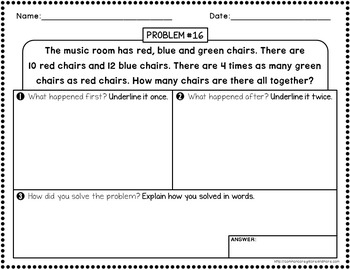DID YOU KNOW:
Seamlessly assign resources as digital activities

Learn how in 5 minutes with a tutorial resource. Try it Now

Learn More# Multi-Step Word Problems - 3rd GradeGrade Levels
2nd - 4th, Homeschool
Subjects
Standards
Resource Type
Formats Included
• PDF
Pages
26 pages

### Description

Multi-Step Word Problems - 3rd Grade (All Four Operations)

This packet contains 24, multi-step word problems where students have to add, subtract, multiply, and divide numbers. Each word problem includes a series of problem solving steps. These steps were created for the purpose of making sense of a problem, instead of using tricks and key words to solve. Students must understand what the problem is asking them to do before using strategies to solve.

There are 3 formats for each word problem. Answer key is included.

•••••••••••••••••••••••••••••••••••••••••••••••••••••••••••••••••••••••••••••••••••

**PLEASE NOTE** This product is included in 2 larger bundles. To view these bundles, click the following links:
MATH RESOURCES FOR GRADE 3
3RD GRADE WORD PROBLEMS {GROWING BUNDLE}

•••••••••••••••••••••••••••••••••••••••••••••••••••••••••••••••••••••••••••••••••••

You might also be interested in the following products:
NO PREP Math Printables for the Whole School Year - 3rd Grade
Common Core Math Assessments for 3rd Grade - ALL STANDARDS

•••••••••••••••••••••••••••••••••••••••••••••••••••••••••••••••••••••••••••••••••••

LET'S STAY CONNECTED!
Be the first to know about my new discounts, freebies and product launches:
• Look for the green star near the top of any page within MY STORE and click it to become a follower. You will then receive customized email updates about my store.
Total Pages
26 pages
Answer Key
Included
Teaching Duration
N/A
Report this Resource to TpT
Reported resources will be reviewed by our team. Report this resource to let us know if this resource violates TpT’s content guidelines.

### Standards

to see state-specific standards (only available in the US).
Solve two-step word problems using the four operations. Represent these problems using equations with a letter standing for the unknown quantity. Assess the reasonableness of answers using mental computation and estimation strategies including rounding.
Fluently multiply and divide within 100, using strategies such as the relationship between multiplication and division (e.g., knowing that 8 × 5 = 40, one knows 40 ÷ 5 = 8) or properties of operations. By the end of Grade 3, know from memory all products of two one-digit numbers.
Understand division as an unknown-factor problem. For example, find 32 ÷ 8 by finding the number that makes 32 when multiplied by 8.
Apply properties of operations as strategies to multiply and divide. Examples: If 6 × 4 = 24 is known, then 4 × 6 = 24 is also known. (Commutative property of multiplication.) 3 × 5 × 2 can be found by 3 × 5 = 15, then 15 × 2 = 30, or by 5 × 2 = 10, then 3 × 10 = 30. (Associative property of multiplication.) Knowing that 8 × 5 = 40 and 8 × 2 = 16, one can find 8 × 7 as 8 × (5 + 2) = (8 × 5) + (8 × 2) = 40 + 16 = 56. (Distributive property.)
Determine the unknown whole number in a multiplication or division equation relating three whole numbers. For example, determine the unknown number that makes the equation true in each of the equations 8 × ? = 48, 5 = __ ÷ 3, 6 × 6 = ?.

### Questions & Answers

Teachers Pay Teachers is an online marketplace where teachers buy and sell original educational materials.

More About Us